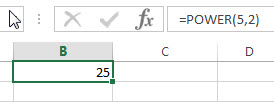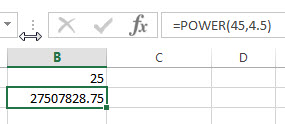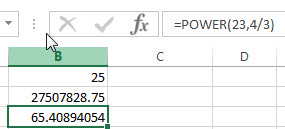# Excel POWER Function

This post will guide you how to use Excel POWER function with syntax and examples in Microsoft excel.

### Description

The Excel POWER function returns the result of a number raised to a given power. So you can use the POWER function to calculate a given number raised to a supplied power in Excel.

The POWER function is a build-in function in Microsoft Excel and it is categorized as a Math and Trigonometry Function.

The POWER function is available in Excel 2016, Excel 2013, Excel 2010, Excel 2007, Excel 2003, Excel XP, Excel 2000, Excel 2011 for Mac.

### Syntax

The syntax of the POWER function is as below:

`= POWER (number,power)`

Where the POWER function argument are:

• number –This is a required argument. The base number that you want to raise to a power
• Power – This is a required argument. The exponent to which the base number is raised.

Note:

• The “^” operator can be used instead of POWER to indicate to what power the base number is to be raised.

### Excel POWER Function Examples

The below examples will show you how to use Excel POWER Function to calculate the result of a number raised to a power.

1# get the result of 5 squared, enter the following formula in Cell B1.

`=POWER(5,2)`2# get the result of number 45 raised to the power of 4.5, enter the following formula in Cell B2.

`=POWER(45,4.5)`3# get the result of number 23 raised to the power of 4/3, enter the following formula in Cell B3.

`=POWER(23,4/3)`